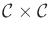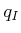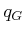There is much less related literature for this chapter in comparison to previous chapters. As explained in Section 8.1, there are historical reasons why feedback is usually separated from motion planning. Navigation functions [541,829] were one of the most influential ideas in bringing feedback into motion planning; therefore, navigation functions were a common theme throughout the chapter. For other works that use or develop navigation functions, see [206,274,750]. The ideas of progress measures , Lyapunov functions (covered in Section 15.1.1), and cost-to-go functions are all closely related. For Lyapunov-based design of feedback control laws, see . In the context of motion planning, the Error Detection and Recovery (EDR) framework also contains feedback ideas .

In , the topological complexity of C-spaces is studied by characterizing the minimum number of regions needed to coverby defining a continuous path function over each region. This indicates limits on navigation functions that can be constructed, assuming that bothandare variables (throughout this chapter,was instead fixed). Further work in this direction includes [326,327].

To gain better intuitions about properties of vector fields,  is a helpful reference, filled with numerous insightful illustrations. A good introduction to smooth manifolds that is particularly suited for control-theory concepts is . Basic intuitions for 2D and 3D curves and surfaces can be obtained from . Other sources for smooth manifolds and differential geometry include [4,107,234,279,872,906,960]. For discussions of piecewise-smooth vector fields, see [27,634,846,998].

Sections 8.4.2 and 8.4.3 were inspired by [235,643] and , respectively. Many difficulties were avoided because discontinuous vector fields were allowed in these approaches. By requiring continuity or smoothness, the subject of Section 8.4.4 was obtained. The material is based mainly on [829,830]. Other work on navigation functions includes [249,651,652].

Section 8.5.1 was inspired mainly by [162,679], and the approach based on neighborhood graphs is drawn from .

Value iteration with interpolation, the subject of Section 8.5.2, is sometimes forgotten in motion planning because computers were not powerful enough at the time it was developed [84,85,582,583]. Presently, however, solutions can be computed for challenging problems with several dimensions (e.g., 3 or 4).Convergence of discretized value iteration to solving the optimal continuous problem was first established in , based on Lipschitz conditions on the state transition equation and cost functional. Analyses that take interpolation into account, and general discretization issues, appear in [168,292,400,565,567]. A multi-resolution variant of value iteration was proposed in . The discussion of Dijkstra-like versions of value iteration was based on [607,946]. The level-set method is also closely related [532,534,533,862].

Steven M LaValle 2020-08-14